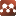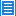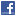## Geometry## A Remark on the Localization Formulas About Two Killing Vector Fields

Authors: Xu Chen

In this article, we will discuss a localization formulas of equlvariant cohomology about two Killing vector fields on the set of zero points ${\rm{Zero}}(X_{M}-\sqrt{-1}Y_{M})=\{x\in M \mid |Y_{M}(x)|=|X_{M}(x)|=0 \}.$ As application, we use it to get formulas about characteristic numbers and to get a Duistermaat-Heckman type formula on symplectic manifold.